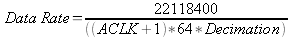The following information is for the EMANT300, EMANT380

# Decimation factor and ACLK value for the ConfigAnalogAdvance function

Question: I am using the ConfigAnalogAdvance function to program the EMANT300 module. And then I am using the ReadAnalog to read a voltage between AIN_COM and AIN_AIN2. Both calls are successful and I am getting a reading. The problem is that even when I electrically connect together the AIN2 with AGND I am reading an unstable voltage, where I should read zero (shorted leads).

I assume that with a 24 bit ADC I should get a very stable reading. My voltage sources are a 9 volt battery with some resistors or a short circuit or a LoadCell. All these sources are known to be very stable. I even shorted the input with a capacitor.

I am still getting a fluctuating signal of about 5 % instability. My suspicion is concerning the Decimation factor and the ACLK values.

Answer: From the guide DAQ with VB2005, the data rate for the ADC is determined by ACLK and Decimation Ratio. First, the ACLK register divides the system clock; that value is then divided by 64 to give us the modulation clock. The data output rate is determined by the Decimation Ratio. The eleven bits in the decimation ratio divide the modulation clock to calculate the data output rate.First ACLK must be between 1 and 127. Once you have chosen the appropriate ACLK value, based on the sampling frequency you will calculate the Decimation factor. However the Decimation factor must less than 2048 (11 bits). If your calculated value if greater, increment the ACLK value by 1 and recalculate the Decimation factor.

If you use a Decimation factor which is 2048 or more you will observe the noise problem you described.